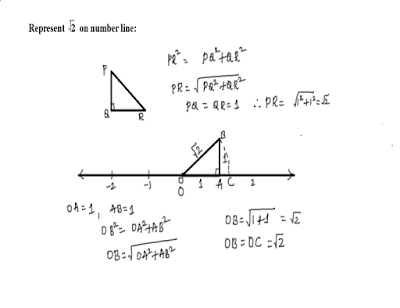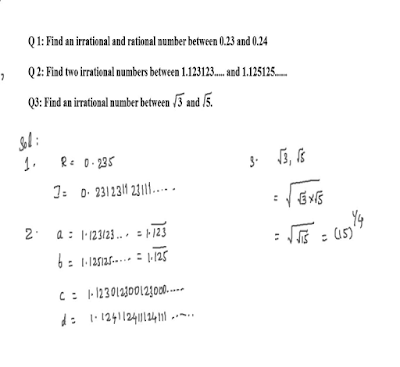Support us .Net Basics C# SQL ASP.NET Aarvi MVC Slides C# Programs Subscribe Download

### Number Systems - Representing Irrational Numbers on number line

Suggested Videos
Part 2 - Number Systems - Terminating Decimals - Text - Slides

In this tutorial we will discuss
• How to represent  irrational numbers on number line
• Inserting an irrational number

Representing Numbers on Number line: To represent a number on number  line follow the steps given below:

1. Draw number line and mark numbers.
2. To represent √2 construct right angle triangle,with base= perpendicular= 1 unit then hypotenuse represents  √2.
3. With O as centre and hypotenuse as radius draw a circle it cuts number line at a point mark this as ‘C’ Distance from ‘O’ to ‘C’  represents √2 i.e OC= √2
4. To represent √3 consider base as √2 and perpendicular= 1 construct right angle triangle  and follow the same steps as above.Inserting An Irrational number:
1. Find an irrational and rational number between 0.23 and 0.24
2. Find two irrational  numbers between 1.123123123…………  and 1.125125125……
3. Find an irrational number between 3 ½ and 5 1/2